# DintDate - to handle dates as easy as you handle simple integers

### Instructions of extension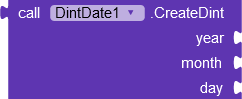``````Creates a dint using given year, month and day.
``````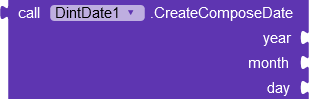``````Creates a dint using any number of years, months and days.
``````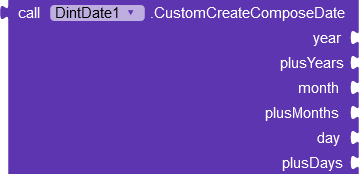``````Creates a dint using any number of years, months and days. but custom
``````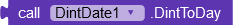``````Return the current date dint.
``````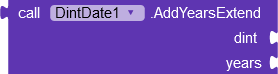``````     * Adds years to a dint. A negative number is allowed.
* If the dint day is the last day in a month then the resulting day will be the last day in a month as well.
````````````Returns a number of days in a given month.
``````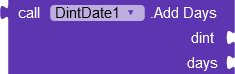``````Adds a given number of days to a given dint. A negative number is allowed.
``````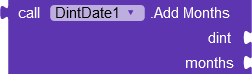``````     * Adds months to a given dint. A negative number is allowed.
* The resulting dint's number of days will be limited by a number of days in the resulting month.
``````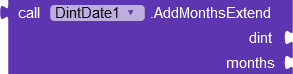``````     * Adds months to a given dint. A negative number is allowed.
* If the dint day is the last day in a month then the resulting day will be the last day in a month as well.
``````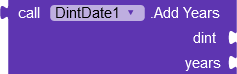``````    * Adds years to a dint. A negative number is allowed.
* The resulting dint's number of days will be limited by a number of days in the resulting month.
``````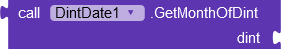``````Returns a month of a given dint.
``````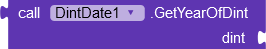``````Returns a year of a given dint.
``````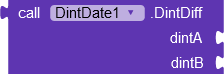``````Returns amount of days between two given dints.
``````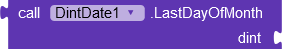``````Returns a dint at the last day of a month of a given dint.
``````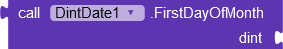``````Returns a dint at the first day of a month of a given dint.
``````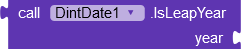``````Returns if a given year is a leap year.
``````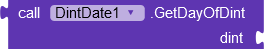``````Returns a day of month of in a given dint.
``````

com.aemo.dintdate.aix (10.1 KB)

If you find my extension useful, please support me

8 Likes

For me, aix file is corrupt after download - no component definition files

2 Likes

Updated it , work now i think

1 Like

Really good extension

1 Like